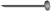# User Forum

Subject :IMO    Class : Class 4

## Ans 1:

Class : Class 5
i did D but they marked it C

Subject :IMO    Class : Class 8

## Ans 1:

Class : Class 8
It is 9

Subject :IMO    Class : Class 4

## Ans 1:

Class : Class 4
You have wrong you have.told that (using one digit at least once)but you have used 5 double time

## Ans 2:

Class : Class 4
you should use the number atleast once. so the greatest no u can make from the numbers provided is 5530. then multiply it with 15. you get the answer.

Subject :IMO    Class : Class 8

Subject :IMO    Class : Class 8

## Ans 1:

Class : Class 8
Constant of proportionality when it is inverse variation is x×y=k So,10×6=60 Find a number which when multiplied by 15 gives 60 60÷15=4 So, and is B - 4

Subject :IMO    Class : Class 3

Class : Class 1

(D): length of crayon = 3length of key = 2Total length of crayon and key =  3+ 2= 5Subject :IMO    Class : Class 2

Class : Class 1

(C): Amount of water Nitesh drinks in one week = 35 litres

= 5+5+5+5+5+5+5
Amount of water Nitesh drinks each day = 5 litres
Amount of water Nitesh drinks in 10 days = 5+5+5+5+5+5+5+5+5+5 = 50 litres.

Subject :IMO    Class : Class 2

Class : Class 1

(A): Govind : 55 > 52
Neha : 96 < 98
Anusha : 86 < 89
Armaan : 98 < 99
So, Govind scored more in Mathematics than in English.

Subject :IMO    Class : Class 2

Class : Class 1

(A): Number of cards John had = 152
Number of cards Karan had = 129
Number of more cards John had than Karan = 152 - 129 = 23

Subject :IMO    Class : Class 2